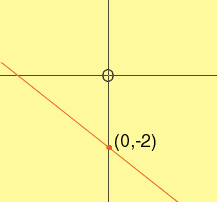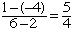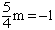Greg secondary level Parent I am helping my son to understand the correct process for two math examples: *If the line with equation 3x-4ky= -16 has y-intercept -2, find k. *Write the equation of the line passing through (2,5) and perpendicular to the line passing through (2,-4) and (6,1) Your help in assisting us would be greatly appreciated. Thanks, Greg Hi Greg, The y-intercept is the y-coordinate of the point where the line crosses the y-axis. Since this is a point on the y-axis, the x-coordinate is zero. In your first problem the y-intercept is -2 and hence the point where the line crosses the y-axis is (0,-2).Since (0,-2) is on the line, (0,-2) satisfies the equation of the line. Thus 3(0) - 4k(-2) = -16 which becomes 8k = -16 and hence k = -2 The fact that you need to solve the second problem is that the products of the slopes of two perpendicular lines is -1. The line through (2,-4) and (6,1) has slopeThus, if the slope of the line you are looking for is m, thenand hence m = -4/5 Finally write the equation of the line through (2,5) with slope -4/5 Cheers, Penny Go to Math Central